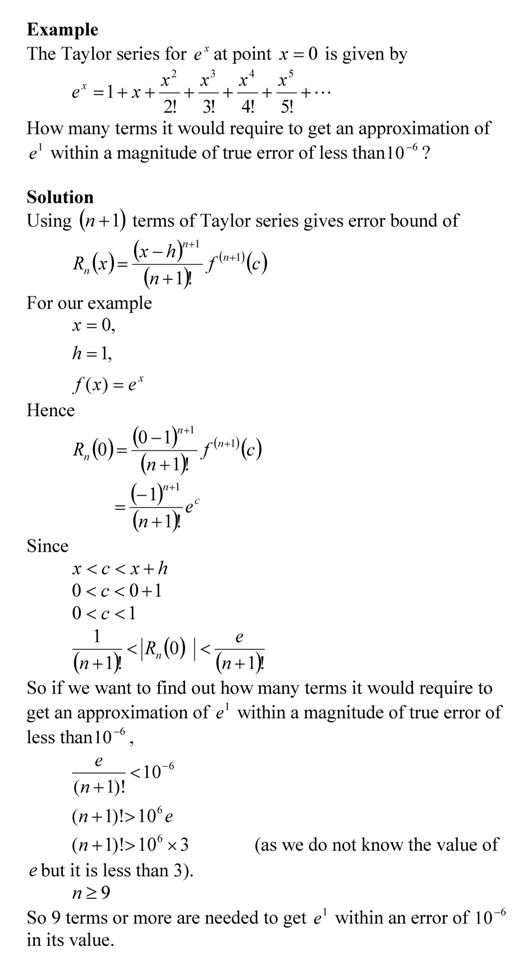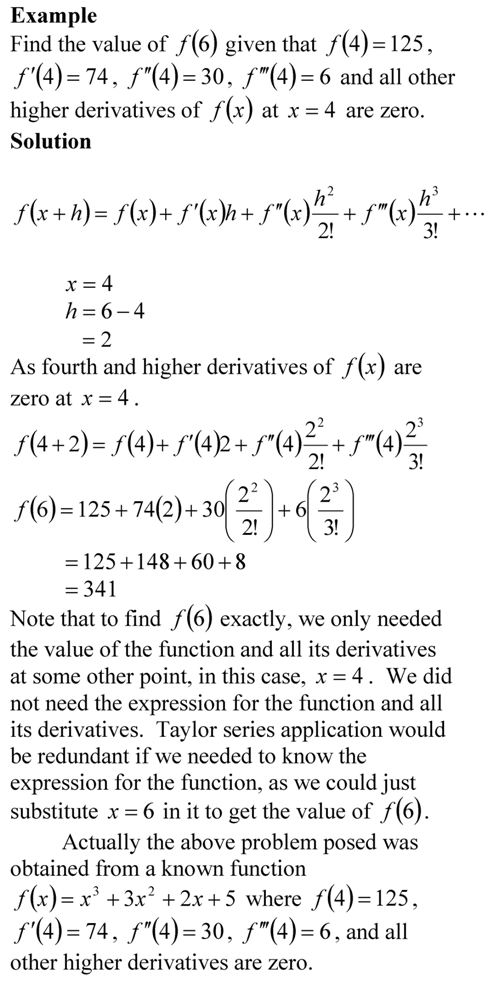## Accuracy of Taylor series

So how many terms should I use in getting a certain pre-determined accuracy in a Taylor series. One way is to use the formula for the Taylor’s theorem remainder and its bounds to calculate the number of terms. This is shown in the example below.This post is brought to you by Holistic Numerical Methods: Numerical Methods for the STEM undergraduate at http://nm.mathforcollege.com.

An abridged (for low cost) book on Numerical Methods with Applications will be in print (includes problem sets, TOC, index) on December 10, 2008 and available at lulu storefront.

Subscribe to the blog via a reader or email to stay updated with this blog. Let the information follow you.

## Taylor series example

If Archimedes were to quote Taylor’s theorem, he would have said, “Give me the value of the function  and the value of all (first, second, and so on) its derivatives at a single point, and I can give you the value of the function at any other point”.

It is very important to note that the Taylor’s theorem is not asking for the expression of the function and its derivatives, just the value of the function and its derivatives at a single point.

Now the fine print: Yes, all the derivatives have to exist and be continuous between x and x+h, the point where you are wanting to calculate the function at. However, if you want to calculate the function approximately by using the nth order Taylor polynomial, then 1st, 2nd,…., nth derivatives need to exist and be continuous in the closed interval [x,x+h], while the (n+1)th derivative needs to exist and be continuous in the open interval (x,x+h).